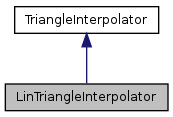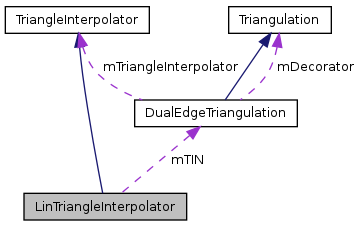QGIS API Documentation  2.0.1-Dufour
LinTriangleInterpolator Class Reference

LinTriangleInterpolator is a class which interpolates linearly on a triangulation. More...

`#include <LinTriangleInterpolator.h>`

Inheritance diagram for LinTriangleInterpolator:[legend]
Collaboration diagram for LinTriangleInterpolator:[legend]

## Public Member Functions

LinTriangleInterpolator ()
Default constructor.
LinTriangleInterpolator (DualEdgeTriangulation *tin)
Constructor with reference to a DualEdgeTriangulation object.
virtual ~LinTriangleInterpolator ()
Destructor.
virtual bool calcNormVec (double x, double y, Vector3D *result)
Calculates the normal vector and assigns it to vec.
virtual bool calcPoint (double x, double y, Point3D *result)
Performs a linear interpolation in a triangle and assigns the x-,y- and z-coordinates to point.
virtual DualEdgeTriangulationgetTriangulation () const
Returns a pointer to the current Triangulation object.
virtual void setTriangulation (DualEdgeTriangulation *tin)
Sets a Triangulation.Public Member Functions inherited from TriangleInterpolator
virtual ~TriangleInterpolator ()

## Protected Member Functions

virtual bool calcFirstDerX (double x, double y, Vector3D *result)
Calculates the first derivative with respect to x for a linear surface and assigns it to vec.
virtual bool calcFirstDerY (double x, double y, Vector3D *result)
Calculates the first derivative with respect to y for a linear surface and assigns it to vec.

## Protected Attributes

DualEdgeTriangulationmTIN

## Detailed Description

LinTriangleInterpolator is a class which interpolates linearly on a triangulation.

Definition at line 24 of file LinTriangleInterpolator.h.

## Constructor & Destructor Documentation

 LinTriangleInterpolator::LinTriangleInterpolator ( )
inline

Default constructor.

Definition at line 51 of file LinTriangleInterpolator.h.

 LinTriangleInterpolator::LinTriangleInterpolator ( DualEdgeTriangulation * tin )
inline

Constructor with reference to a DualEdgeTriangulation object.

Definition at line 56 of file LinTriangleInterpolator.h.

 LinTriangleInterpolator::~LinTriangleInterpolator ( )
inlinevirtual

Destructor.

Definition at line 61 of file LinTriangleInterpolator.h.

## Member Function Documentation

 virtual bool LinTriangleInterpolator::calcFirstDerX ( double x, double y, Vector3D * result )
protectedvirtual

Calculates the first derivative with respect to x for a linear surface and assigns it to vec.

 virtual bool LinTriangleInterpolator::calcFirstDerY ( double x, double y, Vector3D * result )
protectedvirtual

Calculates the first derivative with respect to y for a linear surface and assigns it to vec.

 virtual bool LinTriangleInterpolator::calcNormVec ( double x, double y, Vector3D * result )
virtual

Calculates the normal vector and assigns it to vec.

Implements TriangleInterpolator.

 virtual bool LinTriangleInterpolator::calcPoint ( double x, double y, Point3D * result )
virtual

Performs a linear interpolation in a triangle and assigns the x-,y- and z-coordinates to point.

Implements TriangleInterpolator.

 DualEdgeTriangulation * LinTriangleInterpolator::getTriangulation ( ) const
inlinevirtual

Returns a pointer to the current Triangulation object.

Definition at line 66 of file LinTriangleInterpolator.h.

References mTIN.

 void LinTriangleInterpolator::setTriangulation ( DualEdgeTriangulation * tin )
inlinevirtual

Sets a Triangulation.

Definition at line 71 of file LinTriangleInterpolator.h.

References mTIN.

## Member Data Documentation

 DualEdgeTriangulation* LinTriangleInterpolator::mTIN
protected

Definition at line 44 of file LinTriangleInterpolator.h.

Referenced by getTriangulation(), and setTriangulation().

The documentation for this class was generated from the following file: